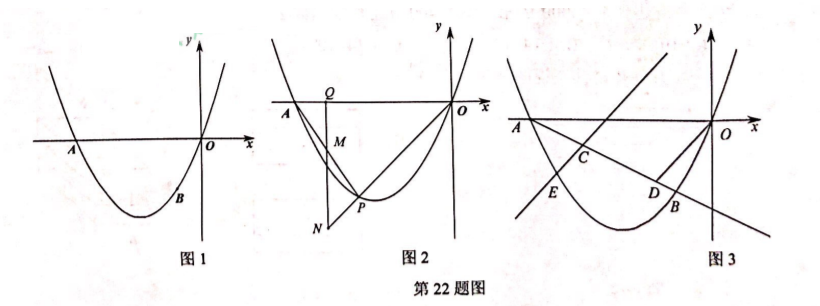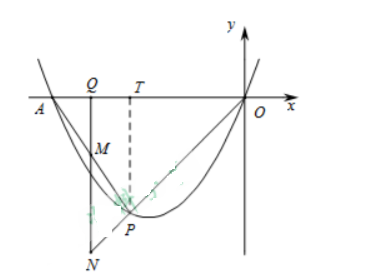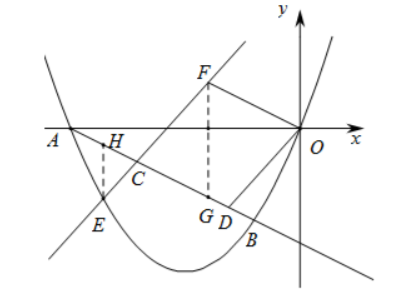(1) 求抛物线解析式;
(2) 如图 2, 点 $P$ 为抛物线上第三象限内一动点, 过点 $Q(-4,0)$ 作 $y$ 轴的平行线, 交直线 $A P$ 于点 $M$, 交直线 $O P$ 于点 $N$, 当点 $P$ 运动时, $4 Q M+Q N$ 的值是否变化? 若变化, 说明变化 规律, 若不变, 求其值;
(3) 如图 3, 长度为 $\sqrt{5}$ 的线段 $C D$ (点 $C$ 在点 $D$ 的左边) 在射线 $A B$ 上移动 (点 $C$ 在线段 $A B$ 上), 连接 $O D$, 过点 $C$ 作 $C E / / O D$ 交抛物线于点 $E$, 线段 $C D$ 在移动的过程中, 直线 $C E$ 经过一定点 $F$, 直接写出定点 $F$ 的坐标与 $\frac{F C}{E C}$ 的最小值.【答案】 解: (1) $\because y=a x^2+b x$ 经过 $A(-5,0), B(-1,-2)$
$$\therefore\left\{\begin{array}{l} 0=(-5)^2 a-5 b \\ -2=(-1)^2 a-b \end{array} \right.$$
$$\therefore\left\{\begin{array}{l} a=\frac{1}{2} \\ \mathrm{~b}=\frac{5}{2} \end{array} \ldots \right.$$
$\therefore$ 抛物线的解析式为 $y=\frac{1}{2} x^2+\frac{5}{2} x$

(2) 过 $P$ 作 $P T / / y$ 轴交 $x$ 轴于点 $T$

$$\because Q(-4,0)$$
$\therefore A Q=1, O Q=4$
$\because N Q / / y$ 轴, $PT // y$ 轴

$\therefore \triangle O T P \subset \triangle \triangle O Q N, \triangle A Q M N \triangle \triangle A T P$
$\therefore \frac{O T}{O Q}=\frac{T P}{Q N}, \frac{A Q}{A T}=\frac{Q M}{T P}$
$\therefore Q N=\frac{T P \cdot O Q}{O T}=\frac{-\frac{1}{2} t^2-\frac{5}{2} t}{-t}=2 t+10$
$Q M=\frac{T P \cdot A Q}{A T}=\frac{-\frac{1}{2} t^2-\frac{5}{2} t}{t+5}=\frac{-t^2-5 t}{2(t+5)}=\frac{4 t}{2}$.

$\therefore 4 Q M+Q N=4 \times \frac{-t}{2}+(2 t+10)=10$(3) 定点 $F(-2,1),$ $\frac{F C}{E C}$ 的最小值是 $\frac{5}{4},$

\begin{aligned} & (-5,0), B(-1,-2) \\ & \therefore\left\{\begin{array} { l } { 0 = - 5 k + m } \\ { - 2 = - k + m } \end{array} \therefore \left\{\begin{array}{l} k=-\frac{1}{2} \\ m=-\frac{5}{2} \end{array}\right.\right. \end{aligned}
$\therefore$ 直线 $A B$ 的解析式为 $y=-\frac{1}{2} x-\frac{5}{2}$
$\because O F / / A B$, 且过 $O(0,0)$$\therefore$ 直线 $O F$ 的解析式为 $y=-\frac{1}{2} x$
$\therefore$ 设 $F\left(n,-\frac{1}{2} n\right)$
$\because C E \| / O D$
$\therefore$ 四边形 $C D O F$ 是平行四边形.
\begin{aligned} & \therefore O F=C D=\sqrt{5} \\ & \therefore n^2+\left(-\frac{1}{2} n\right)^2=(\sqrt{5})^2 \quad \therefore n=\pm 2 \\ & \because n > 0 \\ & \therefore n=-2 \end{aligned}
$\therefore F(-2,1)$ 为直线 $\mathrm{CE}$ 经过的定点.

$\because G$ 在直线 $A B$ 上

\begin{aligned} & \therefore G\left(-2,-\frac{3}{2}\right) \\ & \therefore F G=1-\left(-\frac{3}{2}\right)=\frac{5}{2} \end{aligned}

$$\therefore E H=\left(-\frac{1}{2} t-\frac{5}{2}\right)-\left(\frac{1}{2} t^2+\frac{5}{2} t\right)=-\frac{1}{2} t^2-3 t-\frac{5}{2}=-\frac{1}{2}(t+3)^2+2$$
$\because E H \perp x$ 轴, $F G \perp x$ 轴
$\therefore \triangle E H C \cos \triangle F G C$
$$\therefore \frac{F C}{E C}=\frac{F G}{E H}$$

$\therefore$ 当 $n=-3$ 时, $E H$ 最大值是 2 . 此时 $\frac{F G}{E H}=\frac{5}{4}$
$\therefore \frac{F C}{E C}$ 的最小值是 $\frac{5}{4}$.